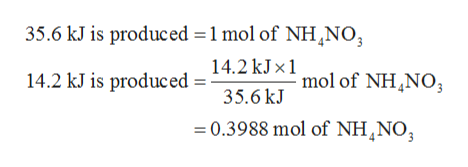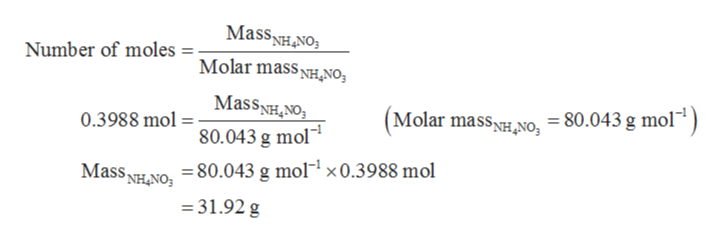# The following thermochemical equation is for the reaction of ammonium nitrate(s) to form N2O(g) and water(g). NH4NO3(s)-> N2O(g)+2H2O(g)         Delta H: -35.6 kJHow many grams of NH4NO3(s) would have to react to produce 14.2kJ of energy?

Question
163 views

The following thermochemical equation is for the reaction of ammonium nitrate(s) to form N2O(g) and water(g).

NH4NO3(s)-> N2O(g)+2H2O(g)         Delta H: -35.6 kJ

How many grams of NH4NO3(s) would have to react to produce 14.2kJ of energy?

check_circle

Step 1

The thermodynamic equation in which ammonium nitrate dissociates to form N2O(g) and water is shown below.

Step 2

From the above reaction 35.6 kJ of energy is being produced from one mole of ammonium nitrate (NH4NO3). The negative sign indicates that the energy is being evolved. Therefore, the number of moles ammonium nitrate (NH4NO3) is required to produce 14.2 kJ of energy is calculated as shown below.help_outlineImage Transcriptionclose35.6 kJ is produced 1 mol of NHNO 14.2 kJx1 mol of NH NO3 14.2 kJ is produced= 35.6 kJ 0.3988 mol of NH NO; fullscreen
Step 3

The mass of ammonium nitrate (NH4NO3) is cal...help_outlineImage TranscriptioncloseMassNHNO Number of moles = Molar massH,NO MassNHNO 80.043 g mol (Molar massHNO, = 80.043 g mol) 0.3988 mol = Mass NHNO80.043 g mol1 x0.3988 mol 31.92 g fullscreen

### Want to see the full answer?

See Solution

#### Want to see this answer and more?

Solutions are written by subject experts who are available 24/7. Questions are typically answered within 1 hour.*

See Solution
*Response times may vary by subject and question.
Tagged in
ScienceChemistry

### Physical Chemistry